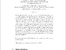# On the Metric Dimension of Corona Product of Graphs

Iswadi, Hazrul and Baskoro, Edy Tri and Simanjuntak, Rinovia (2011) On the Metric Dimension of Corona Product of Graphs. Far East Journal of Mathematical Sciences (FJMS), 52 (2). pp. 155-170. ISSN 0972-0871Preview PDF hazrul_On the Metric Dimension of Corona Product of Graphs_2011.pdf Download (101kB) | Preview
Official URL / DOI: http://pphmj.com/journals/fjms.htm

## Abstract

For an ordered set W = {w_1,w_2,...,w_k} of vertices and a vertex v in a connected graph G, the representation of v with respect to W is the ordered k-tuple r(v|W) = (d(v,w_1),d(v,w_2),...,d(v,w_k)) where d(x,y) represents the distance between the vertices x and y. The set W is called a resolving set for G if every vertex of G has a distinct representation. A resolving set containing a minimum number of vertices is called a basis for G. The metric dimension of G, denoted by dim(G), is the number of vertices in a basis of G. A graph G corona H, G ⊙ H, is de�fined as a graph which formed by taking n copies of graphs H_1,H_2,...,H_n of H and connecting i-th vertex of G to the vertices of H_i. In this paper, we determine the metric dimension of corona product graphs G⊙H, the lower bound of the metric dimension of K_1 +H and determine some exact values of the metric dimension of G⊙H for some particular graphs H.

Item Type: Article Corona graph, metric dimension, resolving set Q Science > QA Mathematics Academic Department > Department of Mathematics and Natural Science Hazrul Iswadi 6179 08 Mar 2012 07:03 20 Mar 2012 01:45 http://repository.ubaya.ac.id/id/eprint/174View Item